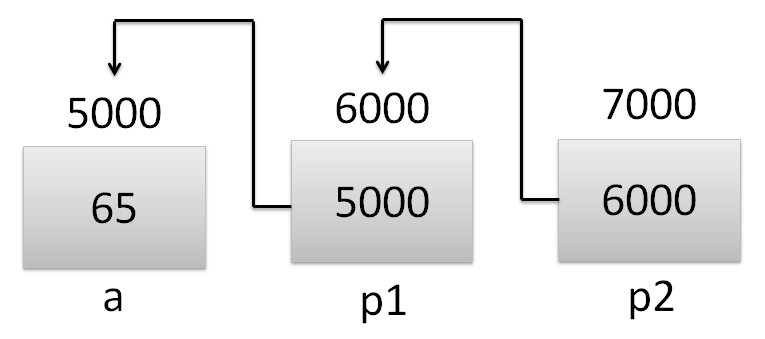# Pointer to Pointer or Double Pointer in C

In this tutorial you will learn about pointer to pointer or double pointer in C.

Pointer is used to store memory address of variable. Double pointer is used to store memory address of any other pointer.

Let’s try to understand this by one example.

Also Read: void pointer in C

## Pointer to Pointer or Double Pointer in CAs you can see in above example that p1 is a pointer as it holds memory address of variable a and p2 is double pointer or pointer to pointer as it holds memory address of pointer p1.

What will be the output if we try to print the values of these 3 variables?

printf(“%d”, p1): 5000 (address of a)

printf(“%d”, *p1): 65 (value of a)

printf(“%d”, p2): 6000 (address of p1)

printf(“%d”, *p2): 5000 (address of a)

printf(“%d”, **p2): 65 (value of a)

Below program will show you how to use double pointer in C language.

Output

a = 65
p1 = 2686744
*p1 = 65
p2 = 2686740
*p2 = 2686744
**p2 = 65

Comment below if you found anything incorrect or have doubts related to above tutorial.

Category: Pointer